# Functions and Function Notation

Definition: A function is any relation (set of ordered pairs) whereby for each element in the domain, there is exactly one element in the range.

Interpretation 1: When a calculation is performed, if there is only ever one possible answer the calculation can be classified as a function.

Example, a function:Findwhen. Answer: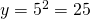Example, not a function:. Findwhen. Answer: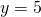or– this relation is not a function. Explicitly,.

Interpretation 2: When looking at a graph, if you put ruler vertically on anyvalue, and if it only every crosses a graph once, the graph represents a function. If it crosses more than once, the graph does not represent a function.

# Function Notation

Suppose we have a relation. We can express the same relation using function notation: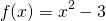A function, like a relation, is a set of ordered pairs. The first element in the ordered pair is the variable, the second element is the value of the expression,. Some ordered pairs generated by this function are:Each function has:

• a name, (in our example,);
• one or more variables (in our example,);
• an expression (in our example,).

# Evaluate: calculate the output

We can define a function by stating an expression and a domain.

Example:Evaluate (a);   (b)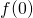;    (c)(a)The ordered pairis a member of this relation.

(b)Zero is usually an easy calculation! The ordered pair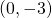is a member of this relation.

(c)Well, 12 is not in the domain. So there is no value forfor this function.

If a domain is not stated, it is assumed that the domain includes all real numbers.

# Practice finding the output

The functions in the following applet are defined for all values of:

A function is well defined when for every input, there is one output.

# Not Well Defined

Consider the function:We would say that this is not a well defined function. It is not well defined as there is a number that does not have an answer – that is,. Better to say:,is a real number but.

or more simply,# Practice finding the input

Sometimes we may have the output of a function and we are required to calculate the input:Findwhen.

In this case, we set up an equation and solve it: